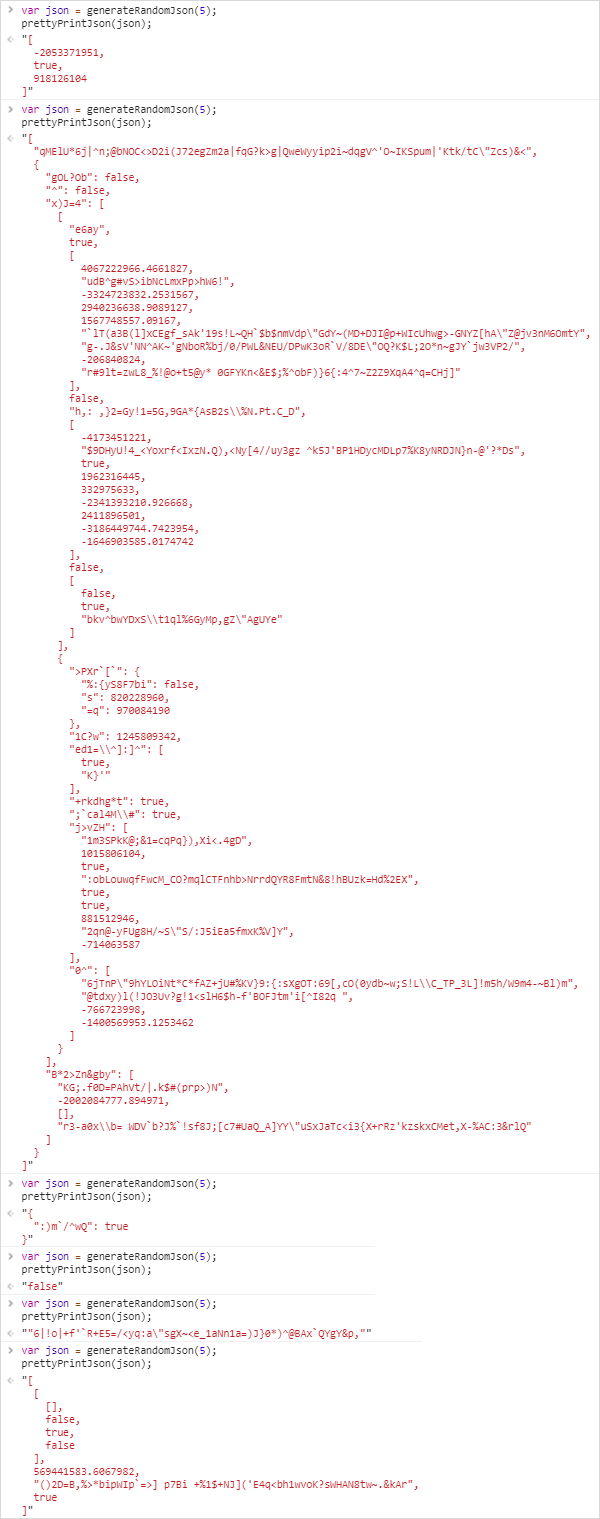Yesterday I wrote a random JSON data structure generator for my next site Online Random Tools. Here's a quick article about what my thought process was when I was writing it.

1. What's a good name for this function? `generateRandomJson` sounds good. Let's put it in code:
```function generateRandomJson () {
```
1. So what is a JSON data structure? It's one of number, string, boolean, array or object. So let's put it in code:
```var choices = ["number", "string", "boolean", "array", "object"];
var choice = chooseOne(choices);

function chooseOne(choices) {
return choices[parseInt(Math.random()*choices.length)];
}
```
1. What is a number? It's an integer or a floating point. Negative or positive. Let's put that in code:
```if (choice == "number") {
return generateRandomNumber();
}

function generateRandomNumber () {
var maxNum = 2**32;
var number = Math.random()*maxNum;
var isInteger = chooseOne([true,false]);
var isNegative = chooseOne([true,false]);

if (isInteger) number = parseInt(number);
if (isNegative) number = -number;

return number;
}
```
1. What is a string? It's zero or more characters. Let's put it in code:
```if (choice == "string") {
return generateRandomString();
}

function generateRandomString () {
var alphabet = " !\"#\$%&'()*+,-./0123456789:;<=>?@ABCDEFGHIJKLMNOPQRSTUVWXYZ[\\]^_`abcdefghijklmnopqrstuvwxyz{|}~";

var maxLength = 100;
var length = Math.random()*maxLength;
var string = "";
for (var i = 0; i < length; i++) {
string += chooseOne(alphabet);
}

return string;
}
```
1. What is a boolean? It's true or false. Let's put it in code:
```if (choice == "boolean") {
return generateRandomBoolean();
}

function generateRandomBoolean () {
return chooseOne([true,false]);
}
```
1. What is an array? It's zero or more of random JSON data structures. Let's put that in code:
```if (choice == "array") {
return generateRandomArray();
}

function generateRandomArray () {
var maxArrayLength = 10;
var length = parseInt(Math.random()*maxArrayLength);

var array = [];
for (var i = 0; i < length; i++) {
array[i] = generateRandomJson();
}

return array;
}
```
1. What is an object? It's zero or more random string keys with random JSON data structures as values. Let's put that in code:
```if (choice == "object") {
return generateRandomObject();
}

function generateRandomObject () {
var maxObjectKeys = 10;
var keyCount = parseInt(Math.random()*maxObjectKeys);

var object = {};
for (var i = 0; i < keyCount; i++) {
var key = generateRandomKeyName();
object[key] = generateRandomJson();
}

return object;
}

function generateRandomKeyName () {
var maxKeyLength = 10;
var keyLength = 1 + parseInt(Math.random()*maxKeyLength);
var randomString = generateRandomString();
return randomString.substr(0,keyLength)
}
```
1. And we're done. We've covered all the cases and can close the `generateRandomJson` function:
```}
```
1. Now let's try it and pretty print it:
```var json = generateRandomJson();
console.log(prettyPrintJson(json));

function prettyPrintJson (json) {
return JSON.stringify(json, null, 2);
}
```

This function will sometimes cause a stack overflow because we haven't limited the depth of the JSON data structure. We can easily do that by passing `maxDepth` as an argument and avoiding generating nested JSON substructures when it's 0. There are many more improvements that can be made, such as passing options for max string length, max number to generate, max number of elements per depth level, etc. I've implemented all these in Random JSON Generator online tool that you can try.

```function generateRandomJson (maxDepth) {
var choices = ["number", "string", "boolean", "array", "object"];
if (maxDepth == 0) {
choices = ["number", "string", "boolean"];
}

var choice = chooseOne(choices);

function chooseOne(choices) {
return choices[parseInt(Math.random()*choices.length)];
}

if (choice == "number") {
return generateRandomNumber();
}
if (choice == "string") {
return generateRandomString();
}
if (choice == "boolean") {
return generateRandomBoolean();
}
if (choice == "array") {
return generateRandomArray();
}
if (choice == "object") {
return generateRandomObject();
}

function generateRandomNumber () {
var maxNum = 2**32;
var number = Math.random()*maxNum;
var isInteger = chooseOne([true,false]);
var isNegative = chooseOne([true,false]);

if (isInteger) number = parseInt(number);
if (isNegative) number = -number;

return number;
}

function generateRandomString () {
var alphabet = " !\"#\$%&'()*+,-./0123456789:;<=>?@ABCDEFGHIJKLMNOPQRSTUVWXYZ[\\]^_`abcdefghijklmnopqrstuvwxyz{|}~";

var maxLength = 100;
var length = Math.random()*maxLength;
var string = "";
for (var i = 0; i < length; i++) {
string += chooseOne(alphabet);
}

return string;
}

function generateRandomBoolean () {
return chooseOne([true,false]);
}

function generateRandomArray () {
var maxArrayLength = 10;
var length = parseInt(Math.random()*maxArrayLength);

var array = [];
for (var i = 0; i < length; i++) {
array[i] = generateRandomJson(maxDepth-1);
}

return array;
}

function generateRandomObject () {
var maxObjectKeys = 10;
var keyCount = parseInt(Math.random()*maxObjectKeys);

var object = {};
for (var i = 0; i < keyCount; i++) {
var key = generateRandomKeyName();
object[key] = generateRandomJson(maxDepth-1);
}

return object;
}

function generateRandomKeyName () {
var maxKeyLength = 10;
var keyLength = 1 + parseInt(Math.random()*maxKeyLength);
var randomString = generateRandomString();
return randomString.substr(0,keyLength)
}
}
```

Now let's generate a random JSON data structure with maxDepth of 5:

```var json = generateRandomJson(5);
prettyPrintJson(json);
```

Success!I also made a nice UI for this tool. You can play with it here:Clear thoughts lead to clear code. See you next time!